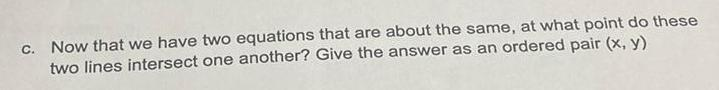Question:

# c Now that we have two equations that are about the same at

Last updated: 9/16/2023c Now that we have two equations that are about the same at what point do these two lines intersect one another Give the answer as an ordered pair x y